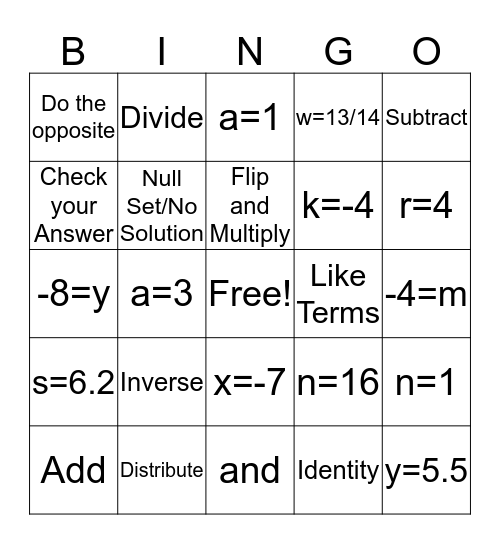# Pre-AlgebraThis bingo card has a free space and 24 words: Inverse, Distribute, Flip and Multiply, Add, Subtract, Do the opposite, Check your Answer, Divide, Like Terms, x=-7, k=-4, -8=y, n=16, n=1, Null Set/No Solution, Identity, -4=m, a=1, r=4, a=3, y=5.5, s=6.2, w=13/14 and and.

## Play Online